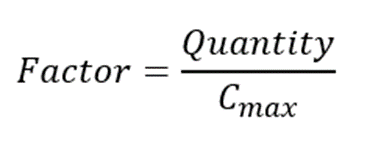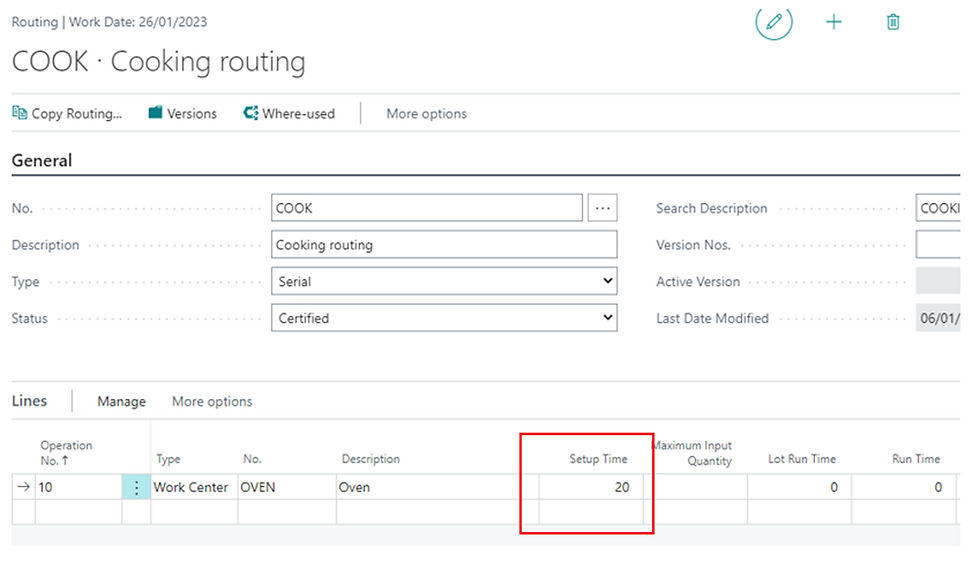top of page
Search
• marco ferrari

# Managing cooking times on routings – A brief custom idea

Updated: Jan 12, 2022

Sometimes in production routings we have to manage situations in which we have a machinery having a limited capacity in input, like for example an oven, that is normally charged with the maximum possible quantity of items in order to optimize production times and costs. In this situation the setup of the routing in Business Central is not possible and we need to make a customization. I will show you an idea for a possible solution.

Suppose for simplicity to have a single operation routing made by an oven having a maximum capacity of 10 pieces with a cooking time of 10 minutes. In this scenario, the total production time depends on how many times we need to put the items into the oven: if the quantity to produce is less than 10, the total time is 10 minutes, but if for example the quantity to produce is just one piece more, 11, then the total time would be 20 minutes. The figure below shows the pointFinally, we can say that the total production time depends on how many times we need to load the oven, that is on the quantity to produce and on the maximum capacity of the oven with this formula:This factor must be rounded to the next upper integer number.

In the standard routing we know that the total production time is given by the sum of the Setup Time plus the Run Time multiplied by the quantity to produce, plus an eventually wait time, so we cannot use the standard times we have on the routing lines, because in our case the run time should be multiplied by the factor above and not by the quantity to produce, as the standard management does.

One possible solution is to add two fields on routing lines, the Maximum Input Quantity and the Lot Run Time.In the example above we have set 10 minutes of Setup Time and the Run Time has been calculated as cooking time for one piece.

Now if we create a production order of 10 pieces on an Item, we will have this final result:As you can see the Run Time has been recalculated as 1 minute for each product, so that the total production time is 20 minutes, the sum of the setup time plus 1 minute multiplied by 10 pieces.

In case of production of 11 pieces we can see that the result will beYou can see that the total time now is 30 minutes (10 minutes of setup time + 10 minutes for the first lot of 10 pieces + 10 minutes for the last piece).

To obtain this result you need to recalculate the Run Time by dividing the total cooking time by the quantity to produce. Below you can see the function I created to make this calculation